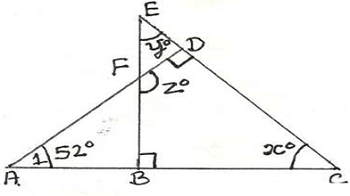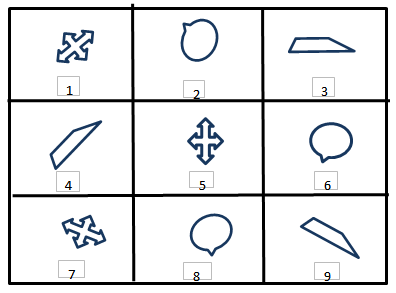# Humming Bird - HMO PDF Sample Papers for Class 10

Class 10 sample paper & practice questions for Humming Bird Mathematics Olympiad (HMO) level 1 are given below. Syllabus for level 1 is also mentioned for these exams. You can refer these sample paper & quiz for preparing for the exam.#### Resources:

##### Sample Questions from Olympiad Success:
 Q.1 Q.2 Q.3 Q.4 Q.5 Q.6 Q.7 Q.8 Q.9 Q.10
 Q.1 A dealer bought 80 cricket bats for ₹ 50 each. He sells 20 of them at a gain of 5%. What must be the gain percentage of the remaining bats, so as to get 10% gain on the whole? a) 3 2/11 % b) 12 1/2 % c) 11 2/3 % d) 4 2/3 %
 Q.2 If f(x) = x² – 5x – 14, find the value of f(7). a) -1 b) 1 c) 0 d) 3
 Q.3 T has child J. J has children M and N. M is married to C and has a child O. What is the relation between O and T? a) mother-in-law b) father-in-law c) grandparent d) great grandparent
 Q.4 Fill in the blank: The value of Polynomial P(x) =2x3 + 6x2 + 5x - 3 at x = -1 is _____. a) -1 b) -2 c) -3 d) -4
 Q.5 6x – 3y + 10 = 0, 2x – y + 9 = 0 represents two lines which are: a) Intersecting at exactly one point b) Intersecting at exactly two points c) Coincident d) Parallel
 Q.6 Find x, y and z in the following figure:a) 38⁰, 52⁰, 142⁰ b) 120⁰, 20⁰, 60⁰ c) 145⁰, 120⁰, 80⁰ d) 45⁰, 120⁰, 80⁰
 Q.7 If 4th and 8th terms of an A.P. are 11 and 23 respectively, find a and d. a) a = 2, d = 3 b) a = 2, d = 2 c) a = 3, d = 3 d) a = 1, d = 3
 Q.8 Identify the identical figures from the following options given.a) (1, 5, 7), (3, 6, 8), (2, 4, 9) b) (1, 4, 7), (2, 6, 8), (3, 5, 9) c) (1, 5, 7), (2, 6, 8), (3, 4, 9) d) (5, 7, 9), (2, 6, 8), (1, 3, 4)
 Q.9 A batsman played three matches in a tournament. The ratio of the score of 1st to 2nd match was 8: 9 and that of the score of 2nd to 3rd match was 3: 2. The difference between the 1st and the 3rd match was 16 runs. What was the batsman's average score in all three matches? a) 40 b) 58 c) 45 1/5 d) 61 1/3
 Q.10 What will be the remainder if f(x) = x³ + 4x² – 3x + 1 is divided by (x – 2)? a) 18 b) 20 c) 19 d) 31Sample PDF of Humming Bird - Humming Bird Mathematics Olympiad (HMO) PDF Sample Papers for Class 10:

 Q.1 )c Q.2 )c Q.3 )d Q.4 )d Q.5 )d Q.6 )a Q.7 )a Q.8 )c Q.9 )d Q.10 )c

Q.1 : c | Q.2 : c | Q.3 : d | Q.4 : d | Q.5 : d | Q.6 : a | Q.7 : a | Q.8 : c | Q.9 : d | Q.10 : c